# 21  Case Study in Cox Regression

## 21.1 Choosing the Number of Parameters and Fitting the Model

• Clinical trial of estrogen for prostate cancer
• Response is time to death, all causes
• Base analysis on Cox proportional hazards model
• $$S(t | X)$$ = probability of surviving at least to time $$t$$ given set of predictor values $$X$$
• $$S(t | X) = S_{0}(t)^{\exp(X\beta)}$$
• Censor time to death at time of last follow-up for patients still alive at end of study (treat survival time for pt.
censored at 24m as 24m+)
• Use simple, partial approaches to data reduction
• Use transcan for single imputation
• Again combine last 2 categories for ekg,pf
• See if we can use a full additive model (4 knots for continuous $$X$$)

A
Predictor Name d.f. Original Levels
Dose of estrogen rx 3 placebo, 0.2, 1.0, 5.0 mg estrogen
Age in years age 3
Weight index: wt(kg)-ht(cm)+200 wt 3
Performance rating pf 2 normal, in bed <50% of time, in bed >50%, in bed always
History of cardiovascular disease hx 1 present/absent
Systolic blood pressure/10 sbp 3
Diastolic blood pressure/10 dbp 3
Electrocardiogram code ekg 5 normal, benign, rhythm disturb., block, strain, old myocardial infarction, new MI
Serum hemoglobin (g/100ml) hg 3
Tumor size (cm$$^2$$) sz 3
Stage/histologic grade combination sg 3
Serum prostatic acid phosphatase ap 3
Bone metastasis bm 1 present/absent
• Total of 36 candidate d.f.
• Impute missings and estimate shrinkage
B
Code
require(rms)
options(prType='html')    # for print, summary, anova
getHdata(prostate)
levels(prostate$ekg)[levels(prostate$ekg) %in%
c('old MI','recent MI')] <- 'MI'
# combines last 2 levels and uses a new name, MI

prostate$pf.coded <- as.integer(prostate$pf)
# save original pf, re-code to 1-4
levels(prostate$pf) <- c(levels(prostate$pf)[1:3],
levels(prostate\$pf))
# combine last 2 levels

w <- transcan(~ sz + sg + ap + sbp + dbp + age +
wt + hg + ekg + pf + bm + hx,
imputed=TRUE, data=prostate, pl=FALSE, pr=FALSE)

attach(prostate)
sz  <- impute(w, sz, data=prostate)
sg  <- impute(w, sg, data=prostate)
age <- impute(w, age,data=prostate)
wt  <- impute(w, wt, data=prostate)
ekg <- impute(w, ekg,data=prostate)

units(dtime) <- 'Month'
S <- Surv(dtime, status!='alive')

f <- cph(S ~ rx + rcs(age,4) + rcs(wt,4) + pf + hx +
rcs(sbp,4) + rcs(dbp,4) + ekg + rcs(hg,4) +
rcs(sg,4) + rcs(sz,4) + rcs(log(ap),4) + bm)
Code
print(f, coefs=FALSE)
Cox Proportional Hazards Model
 cph(formula = S ~ rx + rcs(age, 4) + rcs(wt, 4) + pf + hx + rcs(sbp,
4) + rcs(dbp, 4) + ekg + rcs(hg, 4) + rcs(sg, 4) + rcs(sz,
4) + rcs(log(ap), 4) + bm)

Model Tests Discrimination
Indexes
Obs 502 LR χ2 136.22 R2 0.238
Events 354 d.f. 36 R236,502 0.181
Center -2.9933 Pr(>χ2) 0.0000 R236,354 0.247
Score χ2 143.62 Dxy 0.333
Pr(>χ2) 0.0000
• Global LR $$\chi^2$$ is 135 and very significant $$\rightarrow$$ modeling warranted
• AIC on $$\chi^2$$ scale = $$136.2 - 2 \times 36 = 64.2$$
• Rough shrinkage: 0.74 ($$\frac{136.2 - 36}{136.2}$$)
• Informal data reduction (increase for ap)
CD
Data reduction strategy
Variables Reductions d.f. Saved
wt Assume variable not important enough for 4 knots; use 3 1
pf Assume linearity 1
hx,ekg Make new 0,1,2 variable and assume linearity: 2=hx and ekg not normal or benign, 1=either, 0=none 5
sbp,dbp Combine into mean arterial bp and use 3 knots: map=$$\frac{2}{3}$$ dbp $$+ \frac{1}{3}$$ sbp 4
sg Use 3 knots 1
sz Use 3 knots 1
ap Look at shape of effect of ap in detail, and take log before expanding as spline to achieve stability: add 1 knot -1
Code
heart <- hx + ekg %nin% c('normal','benign')
label(heart) <- 'Heart Disease Code'
map   <- (2*dbp + sbp)/3
label(map) <- 'Mean Arterial Pressure/10'
dd <- datadist(dd, heart, map)

f <- cph(S ~ rx + rcs(age,4) + rcs(wt,3) + pf.coded +
heart + rcs(map,3) + rcs(hg,4) +
rcs(sg,3) + rcs(sz,3) + rcs(log(ap),5) + bm,
x=TRUE, y=TRUE, surv=TRUE, time.inc=5*12)
print(f, coefs=FALSE)
Cox Proportional Hazards Model
 cph(formula = S ~ rx + rcs(age, 4) + rcs(wt, 3) + pf.coded +
heart + rcs(map, 3) + rcs(hg, 4) + rcs(sg, 3) + rcs(sz, 3) +
rcs(log(ap), 5) + bm, x = TRUE, y = TRUE, surv = TRUE, time.inc = 5 *
12)

Model Tests Discrimination
Indexes
Obs 502 LR χ2 118.37 R2 0.210
Events 354 d.f. 24 R224,502 0.171
Center -2.4307 Pr(>χ2) 0.0000 R224,354 0.234
Score χ2 125.58 Dxy 0.321
Pr(>χ2) 0.0000
Code
# x, y for predict, validate, calibrate;
# surv, time.inc for calibrate
anova(f)
 χ2 d.f. P Wald Statistics for S rx 8.01 3 0.0459 age 13.84 3 0.0031 Nonlinear 9.06 2 0.0108 wt 8.21 2 0.0165 Nonlinear 2.54 1 0.1110 pf.coded 3.79 1 0.0517 heart 23.51 1 <0.0001 map 0.04 2 0.9779 Nonlinear 0.04 1 0.8345 hg 12.52 3 0.0058 Nonlinear 8.25 2 0.0162 sg 1.64 2 0.4406 Nonlinear 0.05 1 0.8304 sz 12.73 2 0.0017 Nonlinear 0.06 1 0.7990 ap 6.51 4 0.1639 Nonlinear 6.22 3 0.1012 bm 0.03 1 0.8670 TOTAL NONLINEAR 23.81 11 0.0136 TOTAL 119.09 24 <0.0001
• Savings of 12 d.f.
• AIC=70, shrinkage 0.80
E

## 21.2 Checking Proportional Hazards

• This is our tentative model
• Examine distributional assumptions using scaled Schoenfeld residuals
• Complication arising from predictors using multiple d.f.
• Transform to 1 d.f. empirically using $$X\hat{\beta}$$
• cox.zph does this automatically
• Following analysis approx. since internal coefficients estimated
Code
z <- predict(f, type='terms')
# required x=T above to store design matrix
f.short <- cph(S ~ z, x=TRUE, y=TRUE)
# store raw x, y so can get residuals
• Fit f.short has same LR $$\chi^2$$ of 118 as the fit f, but with falsely low d.f.
• All $$\beta=1$$
F
Code
phtest <- cox.zph(f, transform='identity')
phtest
                   chisq df    p
rx              4.07e+00  3 0.25
rcs(age, 4)     4.27e+00  3 0.23
rcs(wt, 3)      2.22e-01  2 0.89
pf.coded        5.34e-02  1 0.82
heart           4.95e-01  1 0.48
rcs(map, 3)     3.20e+00  2 0.20
rcs(hg, 4)      5.26e+00  3 0.15
rcs(sg, 3)      1.01e+00  2 0.60
rcs(sz, 3)      3.07e-01  2 0.86
rcs(log(ap), 5) 3.59e+00  4 0.47
bm              2.11e-06  1 1.00
GLOBAL          2.30e+01 24 0.52
Code
plot(phtest)  # plot only the first variable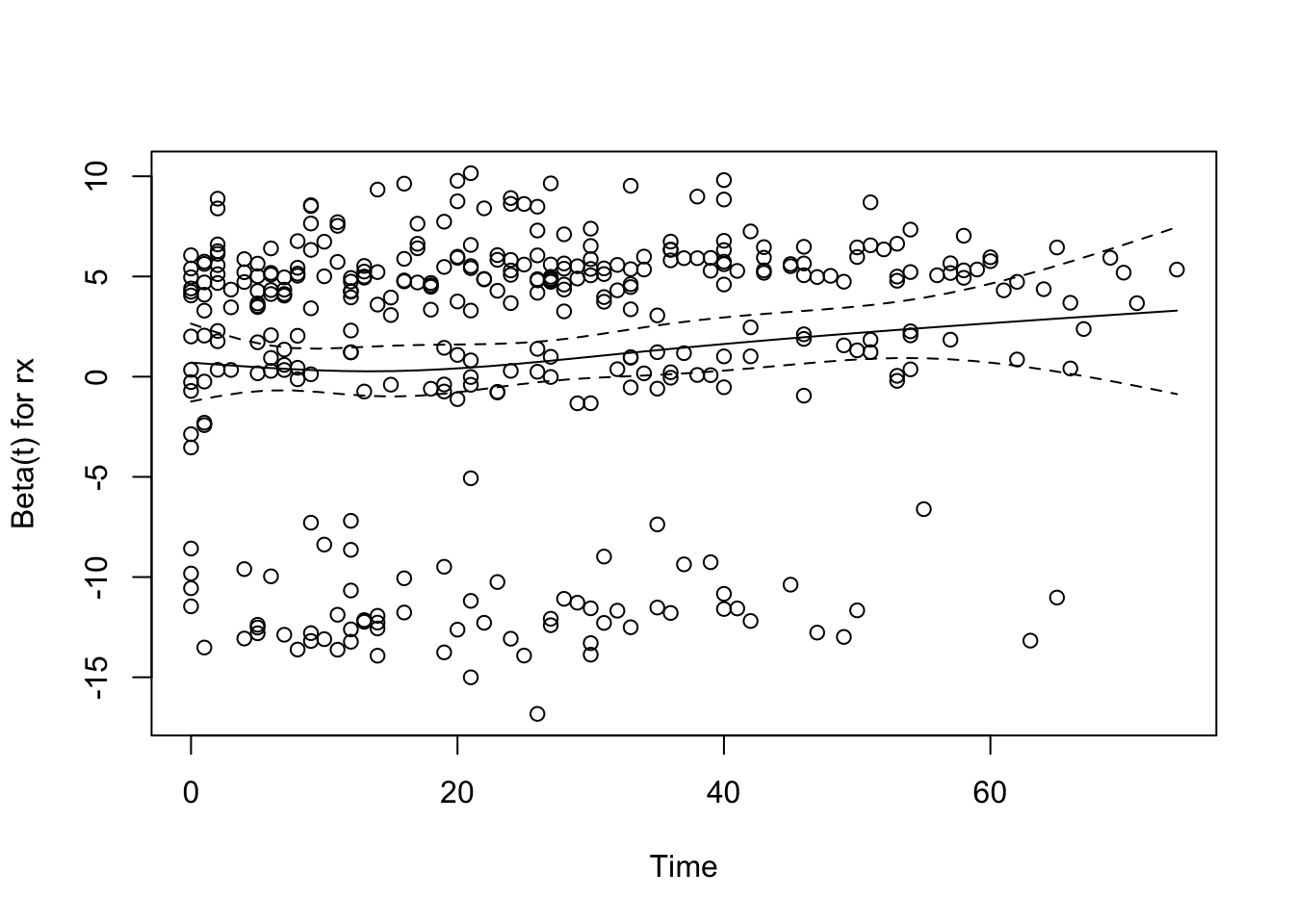Figure 21.1: Raw and spline-smoothed scaled Schoenfeld residuals for dose of estrogen, nonlinearly coded from the Cox model fit, with $$\pm$$ 2 standard errors.
• None of the effects significantly change over time
• Global test of PH $$P=0.52$$

## 21.3 Testing Interactions

• Will ignore non-PH for dose even though it makes sense
• More accurate predictions could be obtained using stratification or time dep. cov.
• Test all interactions with dose
Reduce to 1 d.f. as before
Code
z.dose <- z[,"rx"]  # same as saying z[,1] - get first column
z.other <- z[,-1]   # all but the first column of z
f.ia <- cph(S ~ z.dose * z.other)
anova(f.ia)
 χ2 d.f. P Wald Statistics for S z.dose (Factor+Higher Order Factors) 18.74 11 0.0660 All Interactions 12.17 10 0.2738 z.other (Factor+Higher Order Factors) 125.89 20 <0.0001 All Interactions 12.17 10 0.2738 z.dose × z.other (Factor+Higher Order Factors) 12.17 10 0.2738 TOTAL 129.10 21 <0.0001

## 21.4 Describing Predictor Effects

G
• Plot relationship between each predictor and $$\log \lambda$$
Code
ggplot(Predict(f), sepdiscrete='vertical', nlevels=4,
vnames='names')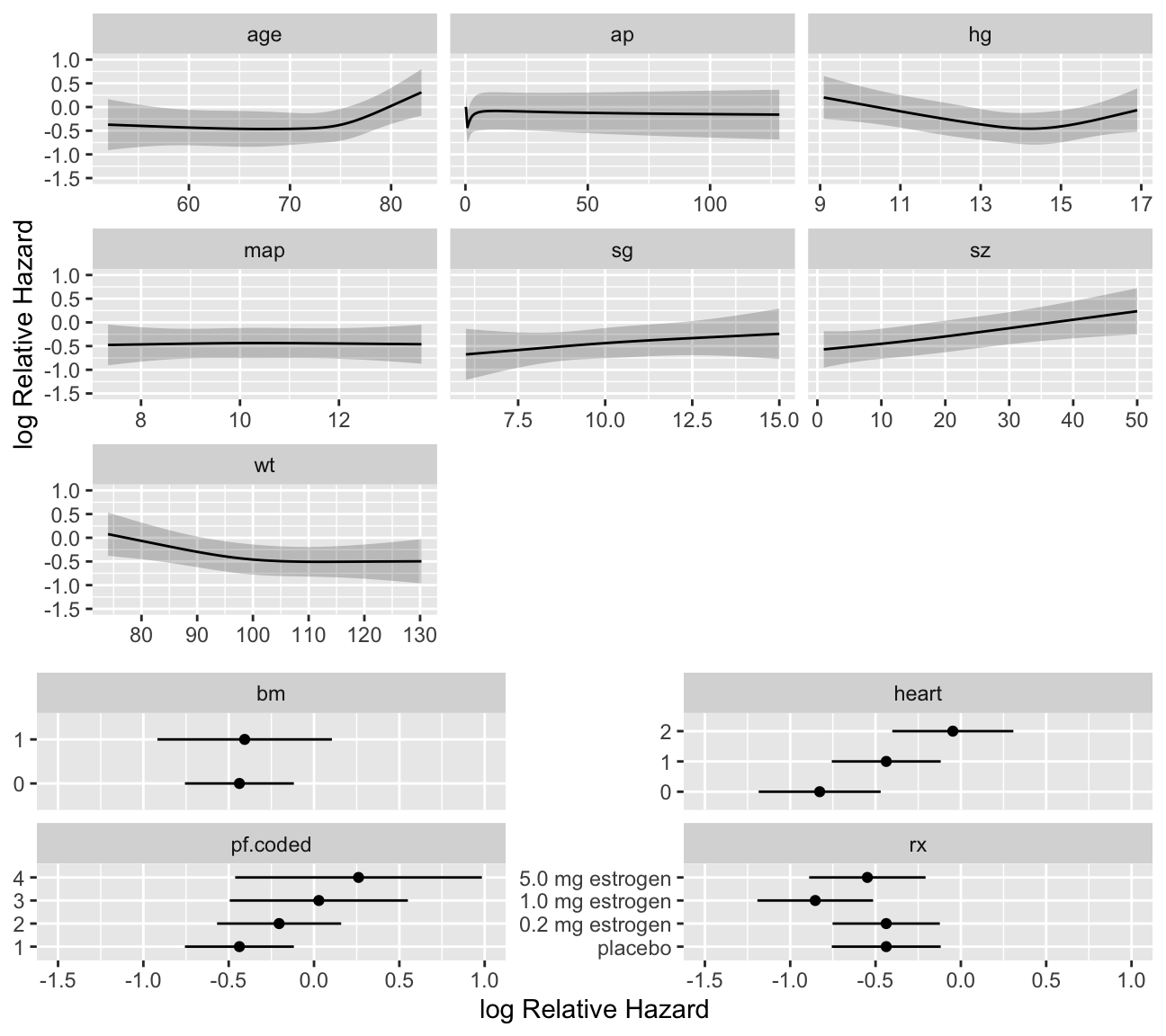Figure 21.2: Shape of each predictor on log hazard of death. $$Y$$-axis shows $$X\hat{\beta}$$, but the predictors not plotted are set to reference values. Note the highly non-monotonic relationship with ap, and the increased slope after age 70 which has been found in outcome models for various diseases.

## 21.5 Validating the Model

H
• Validate for $$D_{xy}$$ and slope shrinkage
Code
set.seed(1)  # so can reproduce results
v <- validate(f, B=300)
html(v)
Index Original
Sample
Training
Sample
Test
Sample
Optimism Corrected
Index
$$n$$
$$D_{xy}$$ 0.3208 0.3494 0.2953 0.0541 0.2667 300
$$R^{2}$$ 0.2101 0.2481 0.1756 0.0724 0.1377 300
Slope 1 1 0.7863 0.2137 0.7863 300
$$D$$ 0.0292 0.0354 0.0239 0.0116 0.0176 300
$$U$$ -5e-04 -5e-04 0.0024 -0.0029 0.0024 300
$$Q$$ 0.0297 0.0359 0.0215 0.0144 0.0153 300
$$g$$ 0.7174 0.7999 0.629 0.1708 0.5466 300
• Shrinkage surprisingly close to heuristic estimate of 0.79
• Now validate 5-year survival probability estimates
Code
cal <- calibrate(f, B=300, u=5*12, maxdim=3)
Using Cox survival estimates at 60 Months
Code
plot(cal)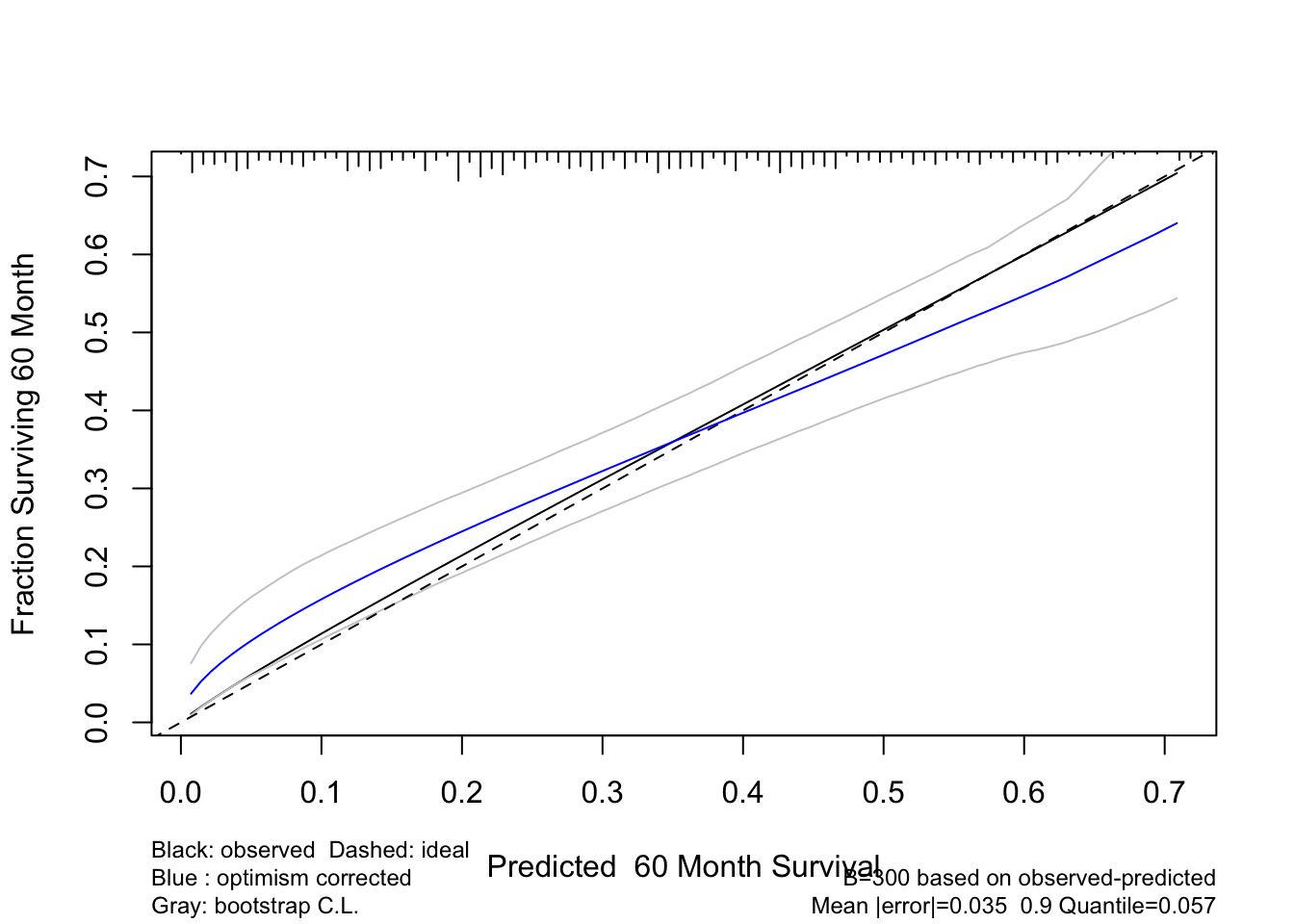Figure 21.3: Bootstrap estimate of calibration accuracy for 5-year estimates from the final Cox model, using adaptive linear spline hazard regression. Line nearer the ideal line corresponds to apparent predictive accuracy. The blue curve corresponds to bootstrap-corrected estimates.

## 21.6 Presenting the Model

• Display hazard ratios, overriding default for ap
Code
spar(top=1)
plot(summary(f, ap=c(1,20)), log=TRUE, main='')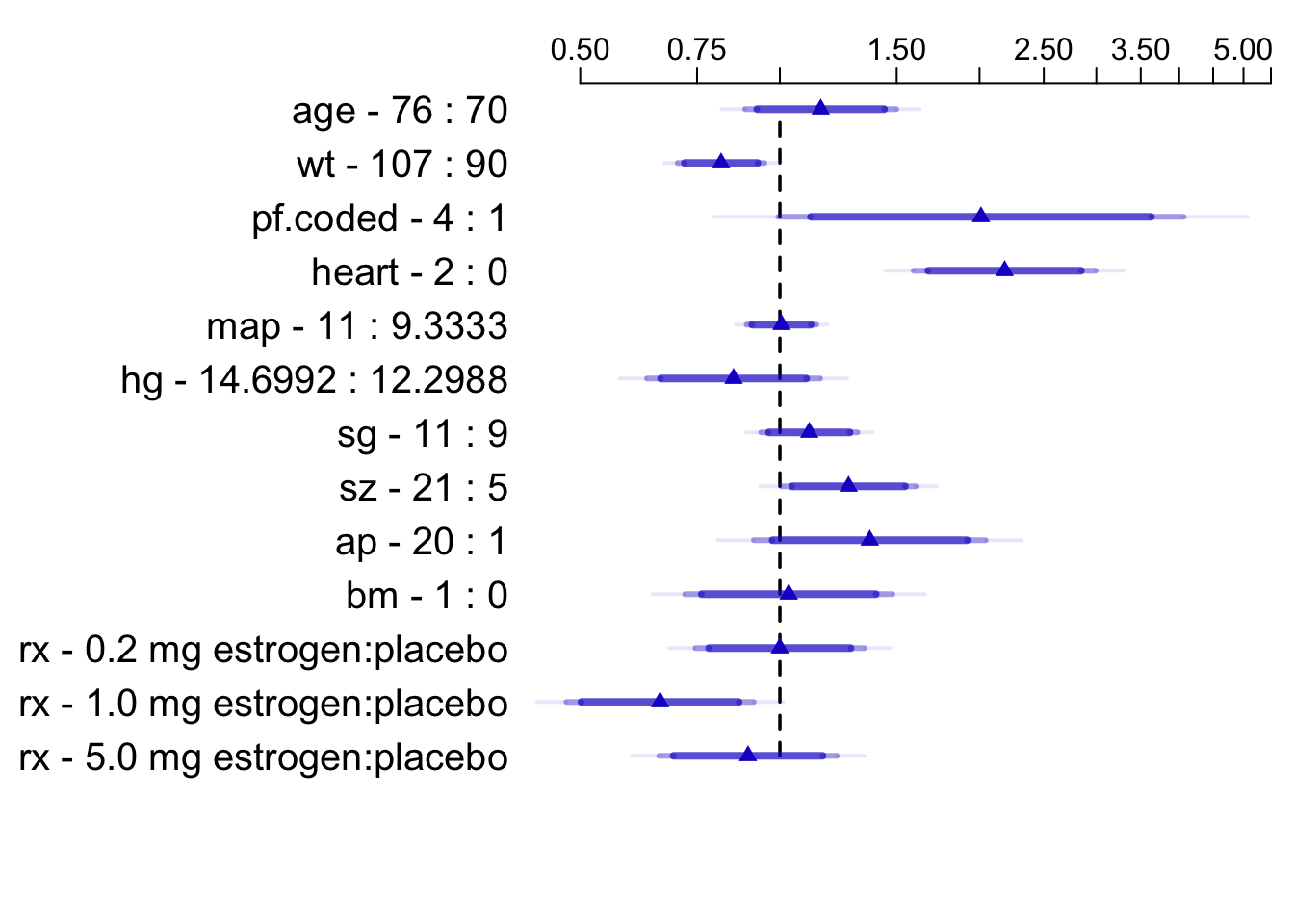Figure 21.4: Hazard ratios and multi-level confidence bars for effects of predictors in model, using default ranges except for ap
• Draw nomogram, with predictions stated 4 ways
Code
spar(ps=8)
surv  <- Survival(f)
surv3 <- function(x) surv(3*12,lp=x)
surv5 <- function(x) surv(5*12,lp=x)
quan  <- Quantile(f)
med   <- function(x) quan(lp=x)/12
ss    <- c(.05,.1,.2,.3,.4,.5,.6,.7,.8,.9,.95)

nom <- nomogram(f, ap=c(.1,.5,1,2,3,4,5,10,20,30,40),
fun=list(surv3, surv5, med),
funlabel=c('3-year Survival','5-year Survival',
'Median Survival Time (years)'),
fun.at=list(ss, ss, c(.5,1:6)))
plot(nom, xfrac=.65, lmgp=.35)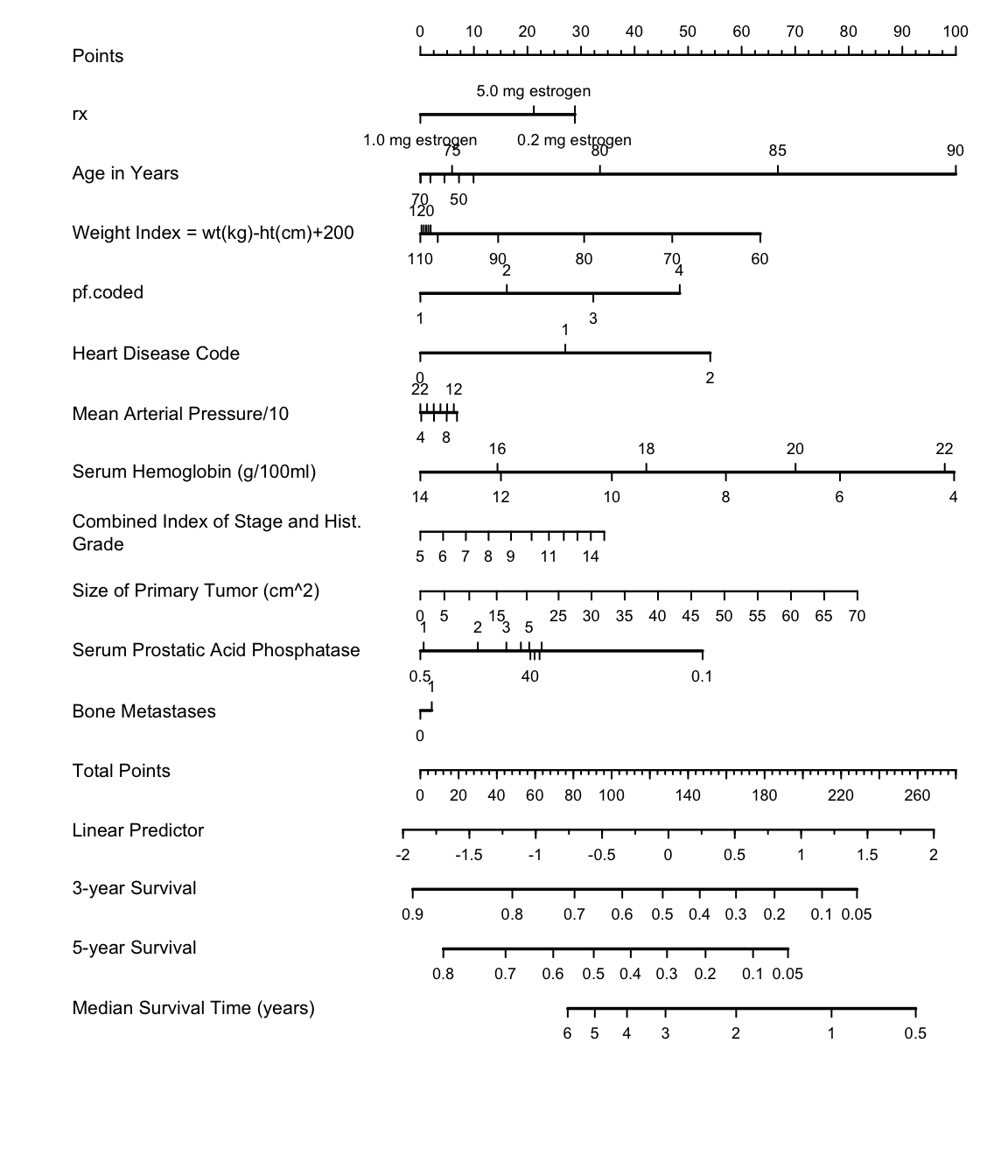Figure 21.5: Nomogram for predicting death in prostate cancer trial